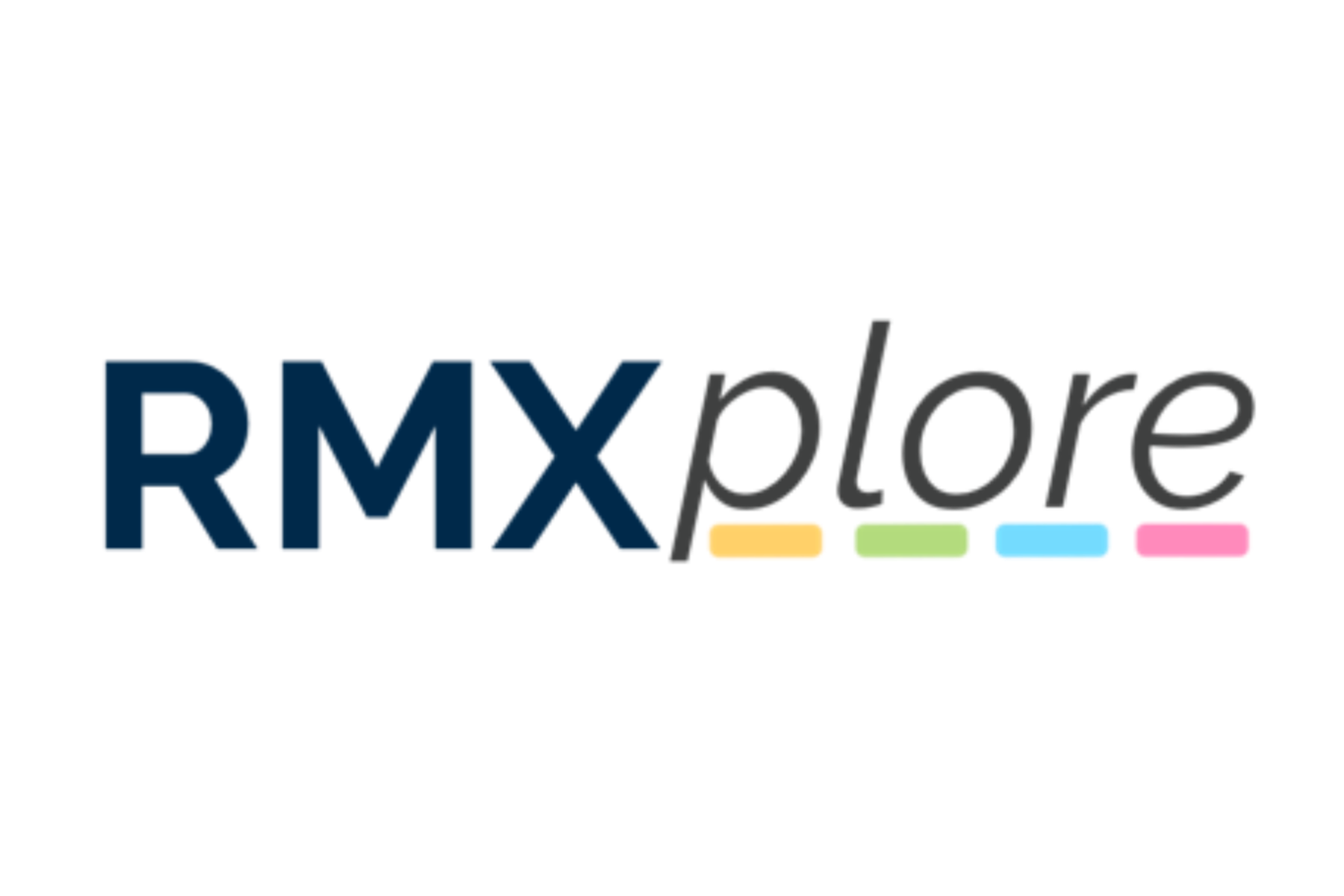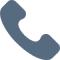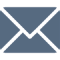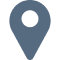# Calculus AB

Please note that RMXplore's Math Bytes Courses are not
currently open for registration
.

### Calculus AB

Coming Soon!
Course Pricing:
• Online : \$260
• Houston Center : \$320
• Cupertino Center : \$400
Course Logistics:
Duration : to be updated
Number of Classes: 5 weeks course
Occurs : to be updated

### Math Byte Course Topics:

Course Topics are organized as six modules with five granular topics covered under each module. Click through below to see all modules/topics for this course.

#### 01 - Limits & Continuity• Definitions and Notation
• Fundamental Derivative Rules (connecting these to the notion of derivatives of a limit)
• Derivatives of Logarithmic and Exponential Functions
• Derivatives of Trigonometric Functions
• Implicit Differentiation

#### 02 - Introduction to Differentiation• Definitions and Notation
• Fundamental Derivative Rules (connecting these to the notion of derivatives of a limit)
• Derivatives of Logarithmic and Exponential Functions
• Derivatives of Trigonometric Functions
• Implicit Differentiation

#### 03 - Applications of Derivatives• Extrema, Graphing, Concavity
• Optimization (word problems)
• Approximations, Linearization, L'Hôpital's Rule
• Related Rates
• Mean / Intermediate Value Theorem

#### 04 - Introduction to Integration• Definition, notion of an integral
• Fundamental Theorem of Calculus - Statement and Intuition
• Applications of the Fundamental Theorem of Calculus
• Calculating Antiderivatives
• Various Riemann sums

#### 05 - Methods of Integration• U-Substitution
• Trigonometric Substitution
• Integration by Parts
• Partial Fraction Decomposition
• Improper Integrals

#### 06 - Definite Integrals and Applications• Definite Integral Manipulations and Simplifications
• Areas of Bounded Regions
• Volume of Solids and Revolutions
• Arc Length
• Moment of Inertia